# supp .pdf

### File information

Original filename: supp.pdf

This PDF 1.5 document has been generated by LaTeX with hyperref package / pdfTeX-1.40.16, and has been sent on pdf-archive.com on 19/08/2017 at 00:13, from IP address 173.247.x.x. The current document download page has been viewed 244 times.
File size: 2.9 MB (5 pages).
Privacy: public file

supp.pdf (PDF, 2.9 MB)

### Document preview

Montreal AI Sympossium Supplementary
Materials
August 18, 2017

1

GAN cost functions

1.1

Vanilla GAN

Cost Function
E

[log D(x)] +

1.2

E

[log(1 − D(G(z)))]

z∼pz (z)

x∼pdata (x)

Wasserstein GAN

Earth-Mover/Wasserstein Distance
W (Pr , Pg ) =

inf

E

[||x − y||]

γ∈Π(Pr ,Pg ) (x,y)∼γ

where Π(Pr , Pg ) is the set of all joint distributions whose marginals are Pr and
Pg .
Cost Function
sup E [D(x)] − E [D(x)]
D x∼Pr

x∼Pθ

where D is some discriminator that is constrained to be K-Lipschitz and Pθ is
the distribution of a generator parameterized by θ.

1.3

Consensus Optimization

Consensus Term

1
||v(x)||2
2
 
∇f
v(x) =
∇g

L(x) =
where

where f and g are the utility functions of the generator and discriminator in
vanilla GANs, respectively.
1

New Utility Functions
fe = f − γL
ge = g − γL
where fe and ge are the new utility functions under the Consensus Optimization
regime.

2

2

Figures

0.6

0.4
0.3

0.4

0.2

0.2

0.1

0.0

0.0
−0.1

−0.2

−0.2

0.6

0.4
0.3

0.4

0.2

0.2

0.1

0.0

0.0
−0.1

−0.2

−0.2

0.6

0.5
0.4

0.4

0.3
0.2

0.2

0.1

0.0

0.0
−0.1

−0.2

−0.2

0.6

0.35
0.30
0.25
0.20
0.15
0.10
0.05
0.00
−0.05
−0.10

0.4
0.2
0.0
−0.2
0.6

0.5
0.4

0.4

0.3
0.2

0.2

0.1

0.0

0.0
−0.1

−0.2

−0.2

0.6

0.4
0.3

0.4

0.2

0.2

0.1

0.0

0.0
−0.1

−0.2

−0.2

0.6

0.4
0.3

0.4

0.2

0.2

0.1

0.0

0.0

−0.2

−0.1

0.6

0.4
0.3

0.4

0.2

0.2

0.1

0.0

0.0
−0.1

−0.2

−0.2
0

50 100150200 0

50 100150200 0

50 100150200 0

50 100150200 0

50 100150200 0

50 100150200 0

50 100150200 0

50 100150200

0

50 100150200 0

(a) Iteration 10000

50 100150200 0

50 100150200 0

50 100150200 0

50 100150200 0

50 100150200 0

50 100150200 0

50 100150200

(b) Iteration 20000

0.5

0.5

0.4
0.3

0.0

0.2

−0.5

0.1
0.0

−1.0

−0.1
0.6
0.5
0.4
0.3
0.2
0.1
0.0
−0.1
−0.2

0.5
0.0
−0.5
−1.0

0.7
0.6
0.5
0.4
0.3
0.2
0.1
0.0
−0.1

0.5
0.0
−0.5
−1.0

0.4

0.5

0.3
0.0

0.2
0.1

−0.5

0.0

−1.0

−0.1
0.5

0.5

0.4
0.3

0.0

0.2

−0.5

0.1
0.0

−1.0

−0.1
0.6
0.5
0.4
0.3
0.2
0.1
0.0
−0.1
−0.2

0.5
0.0
−0.5
−1.0

0.6

0.5

0.5
0.4

0.0

0.3
0.2

−0.5

0.1
0.0

−1.0

−0.1
0.6
0.5
0.4
0.3
0.2
0.1
0.0
−0.1
−0.2

0.5
0.0
−0.5
−1.0
0

50 100150200 0

50 100150200 0

50 100150200 0

50 100150200 0

50 100150200 0

50 100150200 0

50 100150200 0

50 100150200

0

(c) Iteration 30000

50 100150200 0

50 100150200 0

50 100150200 0

50 100150200 0

50 100150200 0

50 100150200 0

50 100150200 0

50 100150200

(d) Iteration 40000

Figure 1: Illustration of Mode Collapse: Each image is a random sample of 64
different walk cycles from the distribution generated by a vanilla GAN, from top
left around clockwise each image is 10000 iterations further into training than
the last. One can see that during training the GAN is jumping from mode to
mode rather than learning to spread out probability mass across the modes.

3

1.0

1.5
1.0
0.5
0.0
−0.5
−1.0
−1.5
−2.0
−2.5

0.5
0.0
−0.5
−1.0
−1.5
−2.0
1.0

1.0

0.5

0.5

0.0

0.0

−0.5

−0.5

−1.0

−1.0

−1.5

−1.5

−2.0

−2.0

−2.5

−2.5

1.5
1.0
0.5
0.0
−0.5
−1.0
−1.5
−2.0
−2.5

1.0
0.5
0.0
−0.5
−1.0
−1.5
−2.0
−2.5

1.0

1.0
0.5
0.0
−0.5
−1.0
−1.5
−2.0
−2.5
−3.0
−3.5

0.5
0.0
−0.5
−1.0
−1.5
−2.0
−2.5
1.0

1.5
1.0
0.5
0.0
−0.5
−1.0
−1.5
−2.0
−2.5
−3.0

0.5
0.0
−0.5
−1.0
−1.5
−2.0
1.0

1.0

0.5

0.5

0.0

0.0

−0.5

−0.5

−1.0

−1.0

−1.5

−1.5

−2.0

−2.0

−2.5

−2.5

1.0

1.0
0.5

0.5

0.0

0.0

−0.5

−0.5

−1.0

−1.0

−1.5

−1.5

−2.0

−2.0

−2.5

1.0

1.5
1.0
0.5
0.0
−0.5
−1.0
−1.5
−2.0
−2.5
−3.0

0.5
0.0
−0.5
−1.0
−1.5
−2.0
−2.5
0

50 100150200 0

50 100150200 0

50 100150200 0

50 100150200 0

50 100150200 0

50 100150200 0

50 100150200 0

50 100150200

(a) Vanilla GAN sample

0

50 100150200 0

50 100150200 0

50 100150200 0

50 100150200 0

50 100150200 0

50 100150200 0

50 100150200 0

50 100150200

(b) Consensus Optimized GAN sample

1.5

1.0

0.5

0.0

−0.5

−1.0

−1.5

−2.0

−2.5

1.0

0.5

0.0

−0.5

−1.0

−1.5

−2.0

−2.5

1.0

0.5

0.0

−0.5

−1.0

−1.5

−2.0

−2.5

1.0

0.5

0.0

−0.5

−1.0

−1.5

−2.0

−2.5

−3.0

−3.5

1.5

1.0

0.5

0.0

−0.5

−1.0

−1.5

−2.0

−2.5

−3.0

1.0

0.5

0.0

−0.5

−1.0

−1.5

−2.0

−2.5

1.0

0.5

0.0

−0.5

−1.0

−1.5

−2.0

−2.5

1.5

1.0

0.5

0.0

−0.5

−1.0

−1.5

−2.0

−2.5

−3.0
0

50

100

150

200

0

50

100

150

200

0

50

100

150

200

0

50

100

150

200

0

50

100

150

200

0

50

100

150

200

0

50

100

150

200

0

50

100

150

200

(d) Conditional GAN sample
(c) Wasserstein GAN sample

4

0.8

1.0

0.6

0.5

0.4

0.0

0.2

−0.5

0.0

−1.0

−0.2

−1.5

−0.4

−2.0

−0.6

−2.5

0.8

1.0

0.6

0.5

0.4

0.0

0.2

−0.5

0.0

−1.0

−0.2

−1.5

−0.4

−2.0

−0.6

−2.5

0.8

1.0

0.6

0.5

0.4

0.0

0.2

−0.5

0.0

−1.0

−0.2

−1.5

−0.4

−2.0

−0.6

−2.5

0.8

1.0

0.6

0.5

0.4

0.0

0.2

−0.5

0.0

−1.0

−0.2
−0.4

−1.5

−0.6

−2.0

0.8

1.0

0.6

0.5

0.4

0.0

0.2

−0.5

0.0

−1.0

−0.2

−1.5

−0.4

−2.0

−0.6

−2.5

0.8

4

0.6

2

0.4

0

0.2

−2

0.0

−4

−0.2

−6

−0.4

−8

−0.6

−10

0.8

4

0.6

2

0.4

0

0.2

−2

0.0

−4

−0.2

−6

−0.4

−8

−0.6

−10

0.8

20

0.6

15

0.4

10

0.2

5

0.0

0

−0.2

−5

−0.4

−10

−0.6

−15
0

50 100150200 0

50 100150200 0

50 100150200 0

50 100150200 0

50 100150200 0

50 100150200 0

50 100150200 0

50 100150200

0

50 100150200 0

(e) Recurrent GAN sample

50 100150200 0

50 100150200 0

50 100150200 0

50 100150200 0

50 100150200 0

50 100150200 0

50 100150200

1.0
0.5
0.0
−0.5
−1.0
−1.5
−2.0
1.0
0.5
0.0
−0.5
−1.0
−1.5
−2.0
−2.5
1.5
1.0
0.5
0.0
−0.5
−1.0
−1.5
−2.0
−2.5
1.0
0.5
0.0
−0.5
−1.0
−1.5
−2.0
1.0
0.5
0.0
−0.5
−1.0
−1.5
−2.0
1.0
0.5
0.0
−0.5
−1.0
−1.5
−2.0
−2.5
1.0
0.5
0.0
−0.5
−1.0
−1.5
−2.0
1.0
0.5
0.0
−0.5
−1.0
−1.5
−2.0
−2.5
0

50 100150200 0

50 100150200 0

50 100150200 0

50 100150200 0

50 100150200 0

50 100150200 0

50 100150200 0

50 100150200

(g) GMM sample
(h) Real sample

Figure 2: Random Samples from generated and real distributions. Random
samples from six different GAN techniques, a GMM baseline, and the real
distribution.

5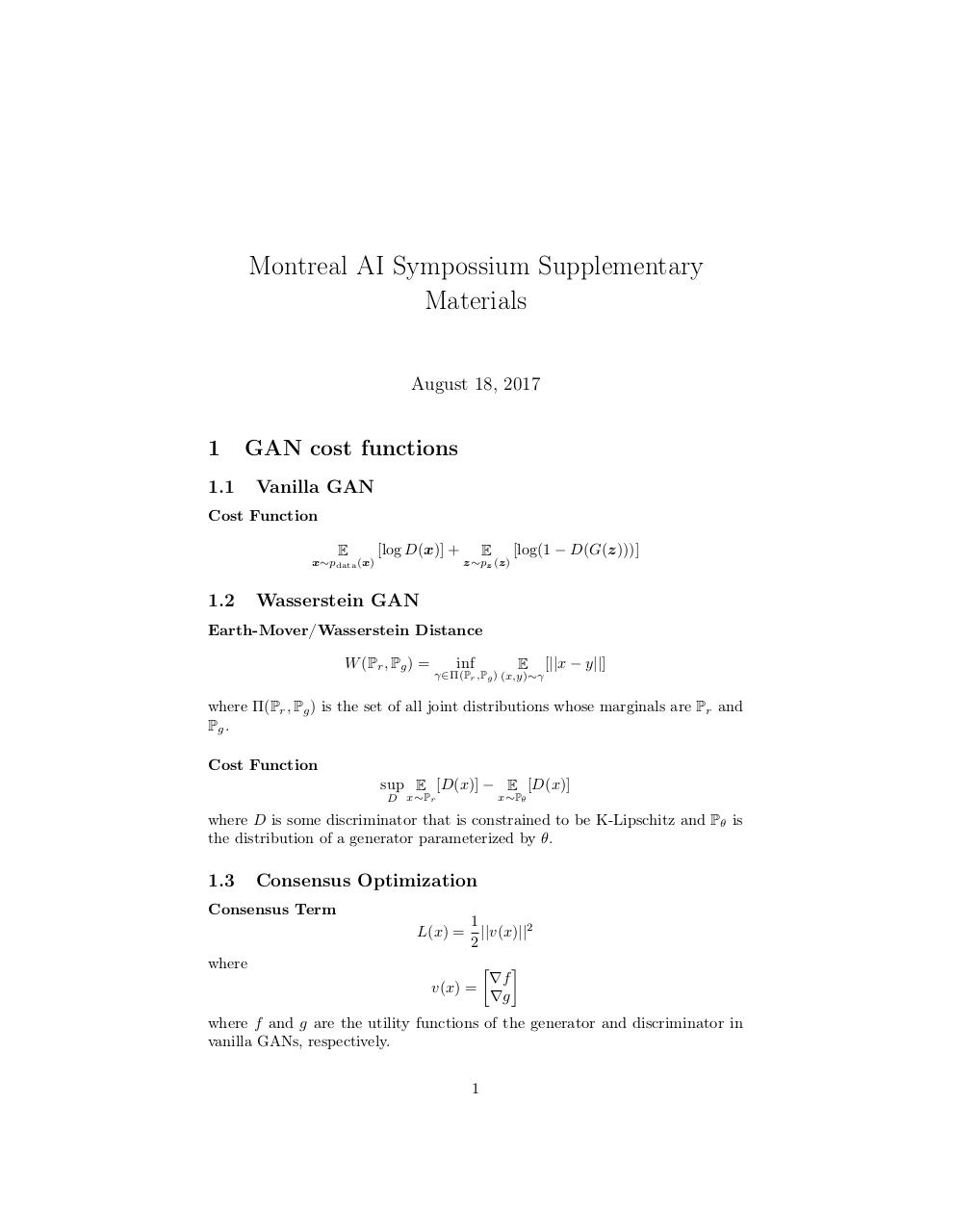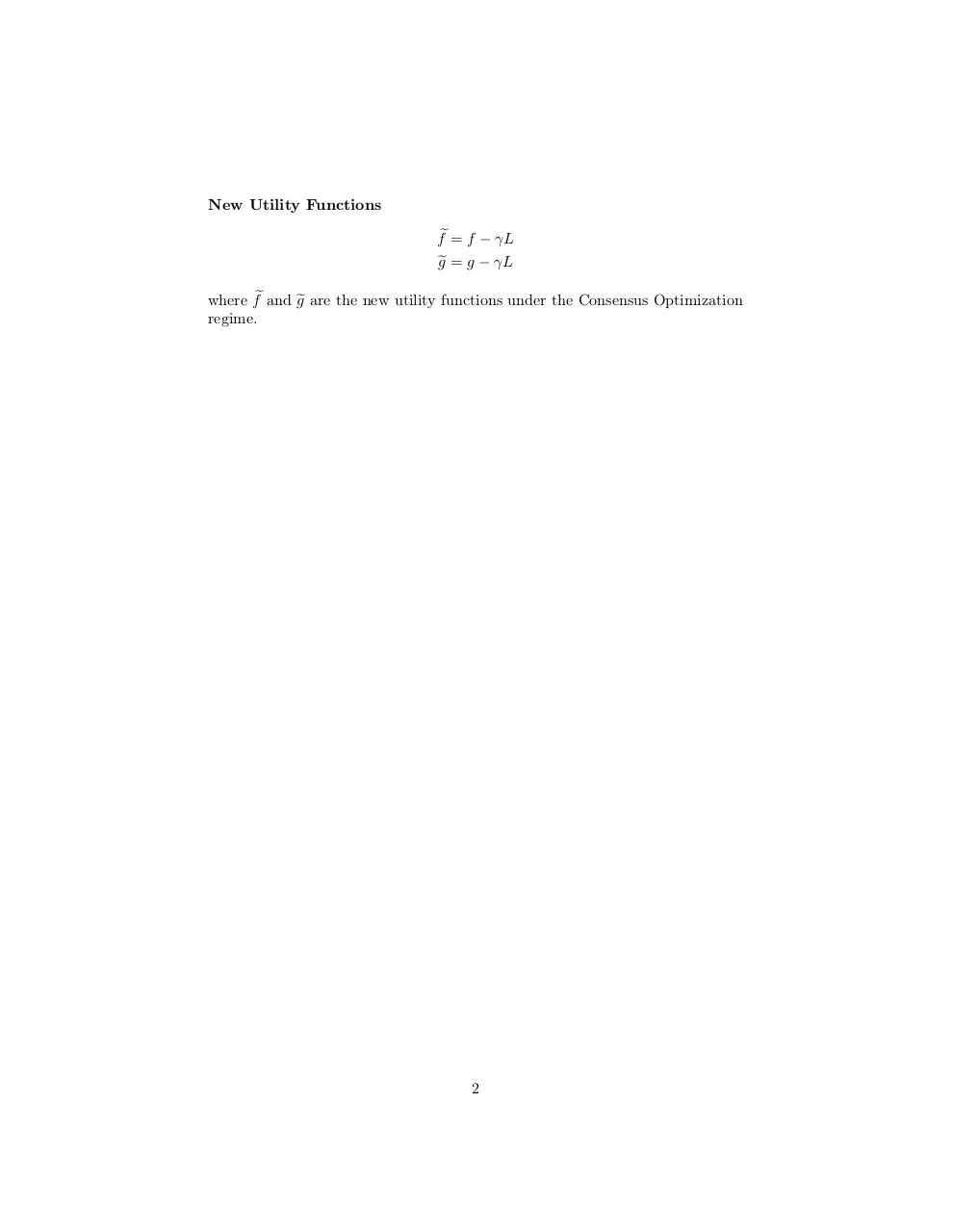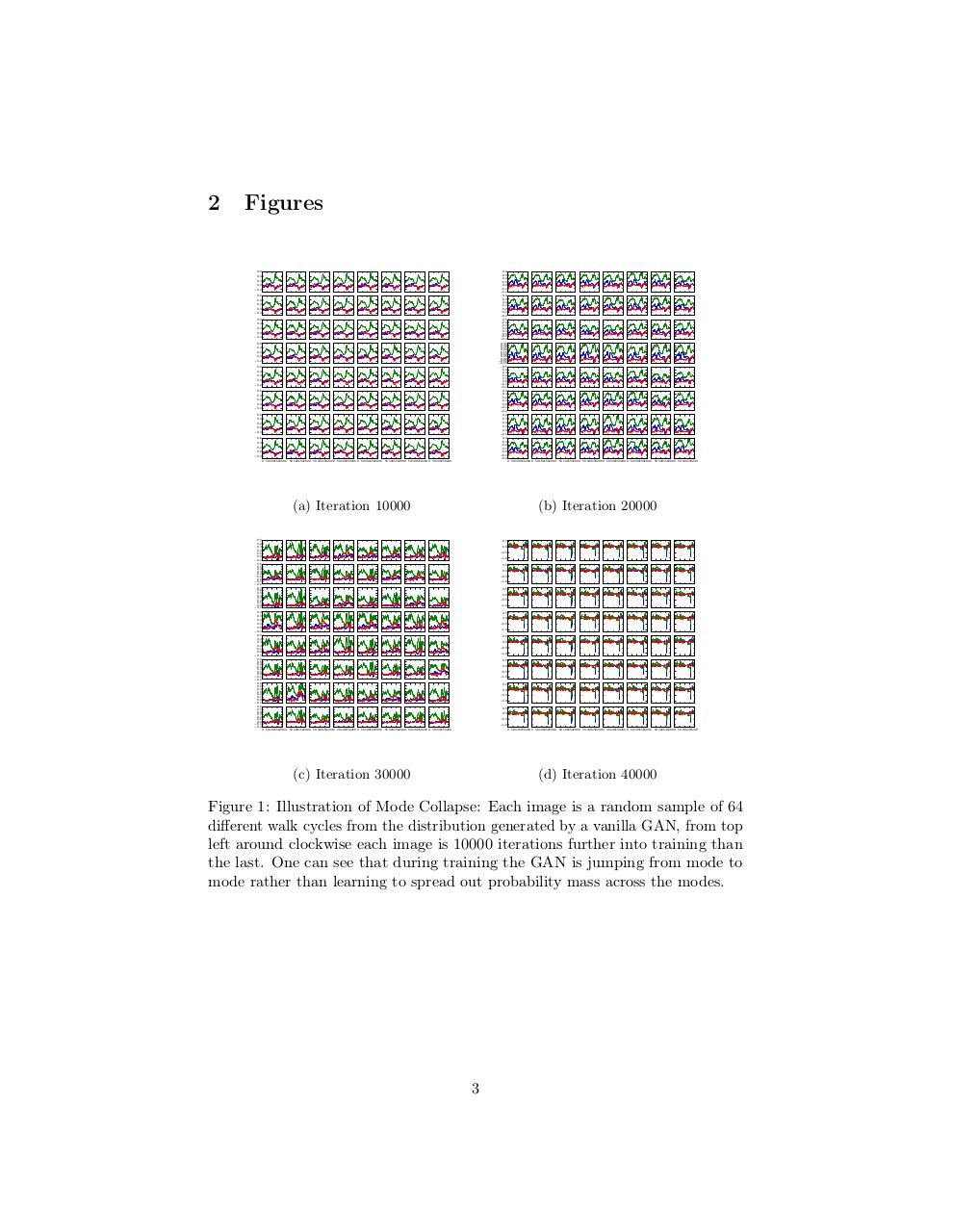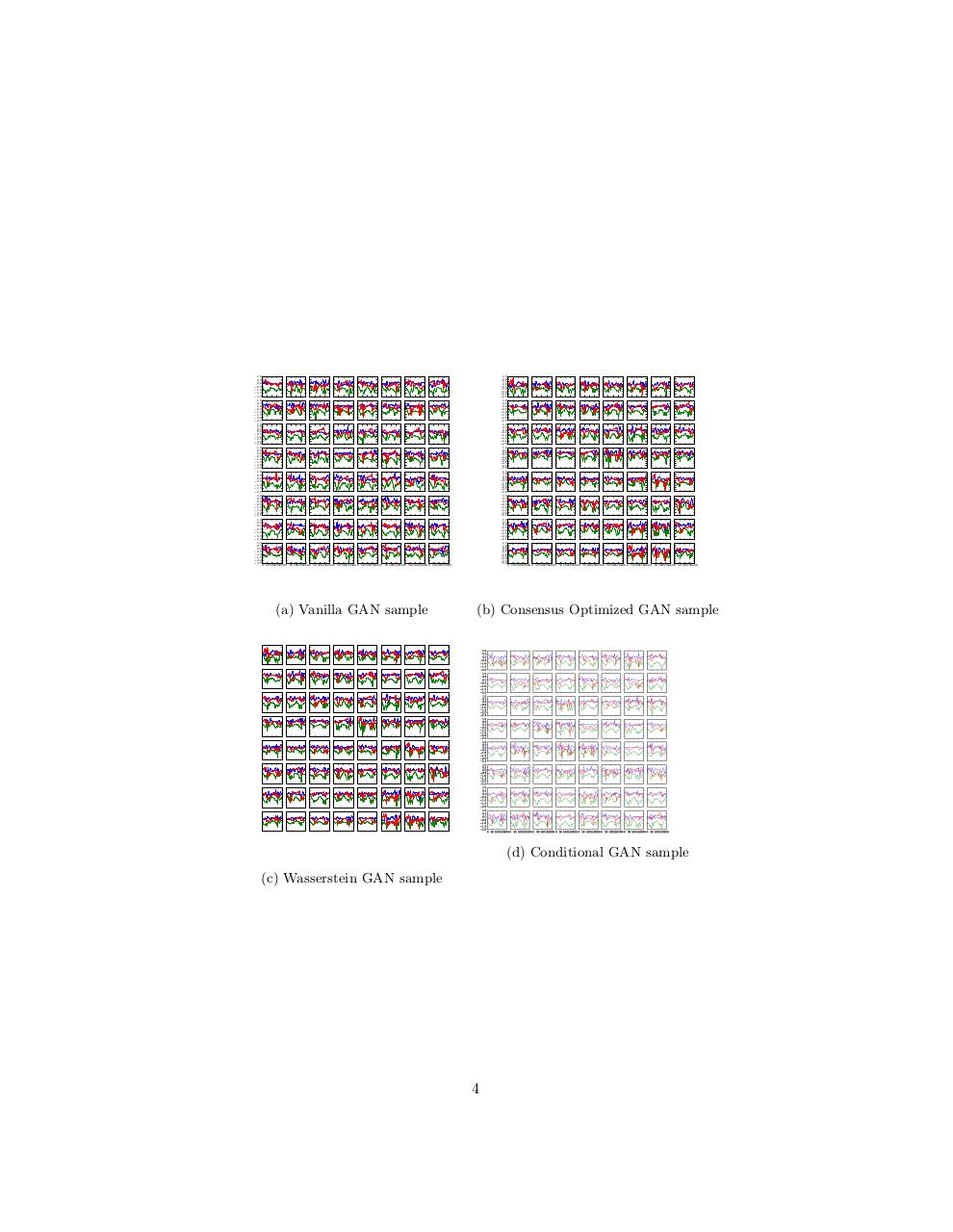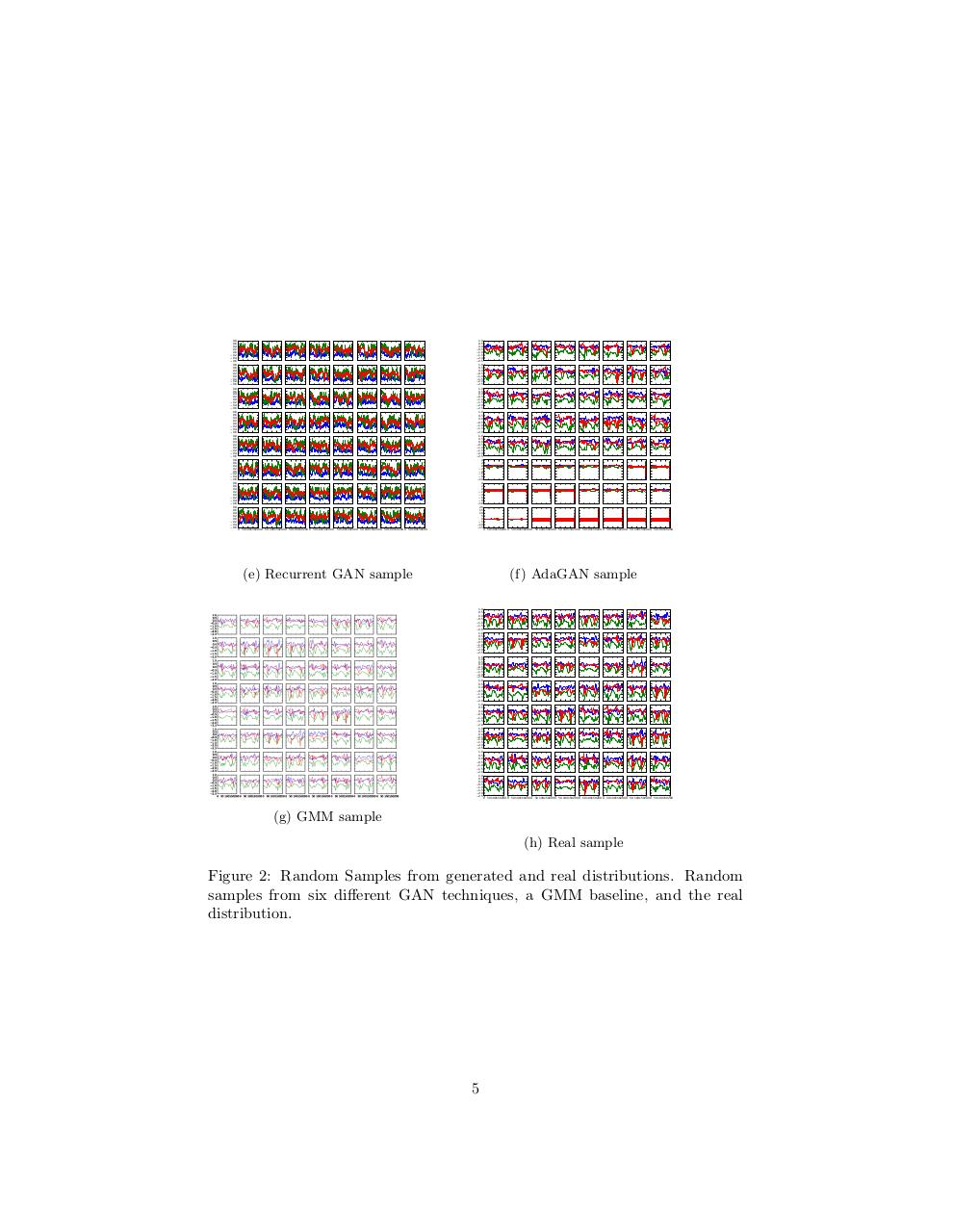#### HTML Code

Copy the following HTML code to share your document on a Website or Blog

#### QR Code### Related keywords